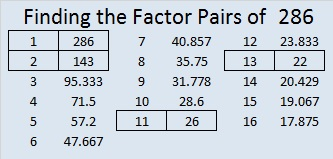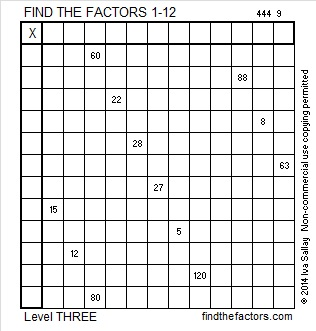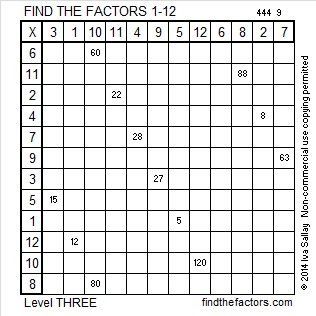# 286 and Level 3

• 286 is a composite number.
• Prime factorization: 286 = 2 x 11 x 13
• The exponents in the prime factorization are 1, 1, and 1. Adding one to each and multiplying we get (1 + 1)(1 + 1)(1 + 1) = 2 x 2 x 2 = 8. Therefore 286 has 8 factors.
• Factors of 286: 1, 2, 11, 13, 22, 26, 143, 286
• Factor pairs: 1 x 286, 2 x 143, 11 x  26, or 13 x 22
• 286 has no square factors that allow its square root to be simplified. √286 ≈ 16.912Print the puzzles or type the factors on this excel file: 12 Factors 2014-11-03

A Logical Approach to FIND THE FACTORS: Find the column or row with two clues and find their common factor. Write the corresponding factors in the factor column (1st column) and factor row (top row).  Because this is a level three puzzle, you have now written a factor at the top of the factor column. Continue to work from the top of the factor column to the bottom, finding factors and filling in the factor column and the factor row one cell at a time as you go.This site uses Akismet to reduce spam. Learn how your comment data is processed.